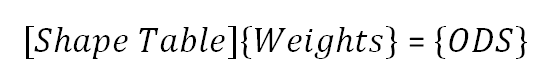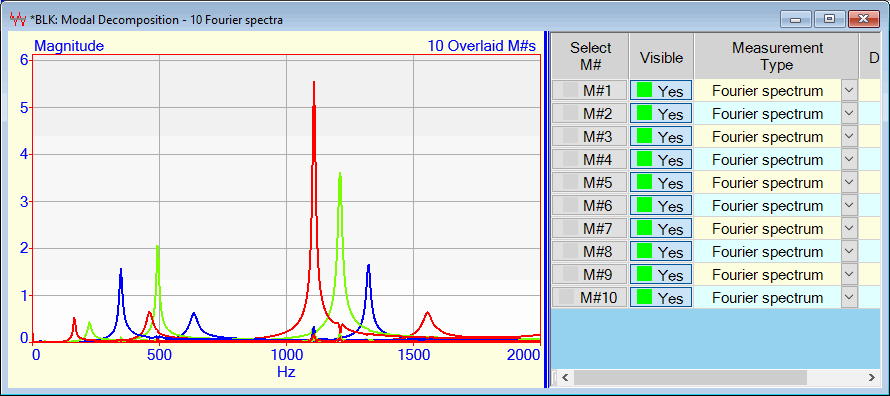# Modes | Modal Decomposition

Decomposes time or frequency measurements into "resonance curves" that represent the contribution of each mode shape or ODS to the measurement data. Solves the following equation for each M#, at each sample of data in a Data Block,where:

[ Shape Table ] = matrix of mode shapes or operating deflection shapes (ODS's).  Each matrix column is a mode shape or ODS.

{ Weights } = vector of weights (also called a participation vector), for each sample of time or frequency data in the Data Block

{ ODS } = the ODS of data at each sample for each sample of time or frequency data in the Data Block

Each resonance curve is saved as a new M# in a new Data Block.  For example, if 10 mode shapes are used to decompose the time or frequency data in a Data Block, 10 M#s, each one a resonance curve for each mode, are calculated.

Only mode shapes are used for Modal Decomposition.
Modal Frequency & Damping are not used.Data Block Showing Modal Decomposition Resonance Curves Overlaid.

Each modal decomposition resonance curve can be curve fit to extract the experimental modal frequency & damping associated with that resonance.